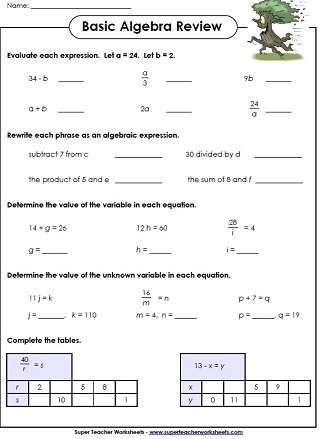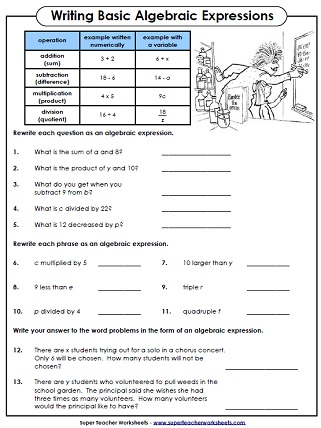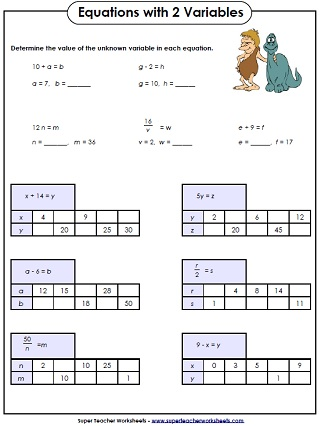# Algebra Worksheets - Basic

These basic algebra worksheets introduce students to using and writing algebraic equations with letter variables.Evaluate each expression; Substitute numbers for variables to solve.
5th through 7th Grades
Write simple expressions in algebraic form with a variable
5th through 7th Grades
Another worksheet in which students are required to find the value of the variables.
5th through 7th Grades
Learn to identify constants, variables, expressions, and equations.
5th through 7th Grades
Determine which algebraic equations are true, and which ones are not.
5th through 7th Grades
Complete the tables by substituting different numbers for variables.
5th through 7th Grades
Choose the best equation to solve each word problem.  Then, calculate the value of the variable.
5th through 7th Grades
Why did the scarecrow win the Nobel Prize? To find out, find the value of x for each algebraic equation. Then match the letters with the numbers in the puzzle.
5th through 7th Grades
Students will solve for one of the two missing variables.
5th through 7th Grades
Students will find the value of a variable when 2 variables are given.
5th through 7th Grades
Mixed review page with different types of basic algebra problems
5th through 7th Grades
Geometry Worksheets

Geometry worksheets for teaching angles, lines, polygons, solid shapes, symmetry, and more

Equations (Basic Algebra)

Practice balancing simple algebraic equations and finding the value of variables.

Sample Images of
Algebra Worksheets